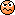# Capacitor Discharge Time Formula Euler!

I would like to understand how the Euler constant elevated to fractional exponent gave this result? 958mV someone please!?Related Introductory Physics Homework Help News on Phys.org
Svein
You did not give enough information for us to check everything, but the calculation is correct (I checked it on my old HP calculator).

It is because my doubt is the fractional exponent, do not know why has this result!

Personal My question is in Euler's constant raised to a fractional exponent!
Why and how ?? I do not know calculate HP, it's good we mastered without having to use HP,

I have a teaching video, but honestly I still do not understand if you want to see the video? I have!

Last edited:
cnh1995
Homework Helper
Gold Member
Charging of a capacitor is an exponential
event. Voltage across capacitor rises exponentially as
Vc=Vf(1-e-t/T). Time t is in the exponent, hence the part e-t/T is responsible for the exponential increase in voltage w.r.t time.

•NickTesla
Svein
OK. Let us take it in steps:
1. $\frac{10}{47}= 0.212765957$
2. $e^{-0.212765957}=0.808345302$
3. $1- 0.808345302=0.191655$
4. $5 \cdot 0.191655 = 0.958273$

•NickTesla
Yes! , I understand, but using numbers,
Watch this video!

OK. Let us take it in steps:
1. $\frac{10}{47}= 0.212765957$
2. $e^{-0.212765957}=0.808345302$
3. $1- 0.808345302=0.191655$
4. $5 \cdot 0.191655 = 0.958273$

OK. Let us take it in steps:
1. $\frac{10}{47}= 0.212765957$
2. $e^{-0.212765957}=0.808345302$
3. $1- 0.808345302=0.191655$
4. $5 \cdot 0.191655 = 0.958273$
Sven Wonderfully Thank you!

Sven Wonderfully Thank you!

Personally, I'll be honest, here in South America (Brazil) I doubt that the Brazilian has a video explaining speaking Neper calculation on youtube to calculate the potentiation, constant = e ^ -0.212765957,
I doubt (I dúvi [do] D + O = DO = DEODO talking!). Kkk! I doubt DEODO is when we doubt very much! is the Brazilian way of speaking! My God there are only understand potentiation, that's all, and I going crazy, studying (Mantissa) studies (Mantissa) kkkk lol (mathematics: decimal part of a logarithm) !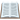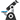(Matematicas: decimal part of a logarithm)! I wanted the help of North America, if possible help from the American continent LOL kkkk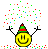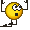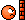how does it do??